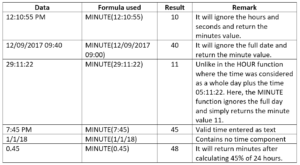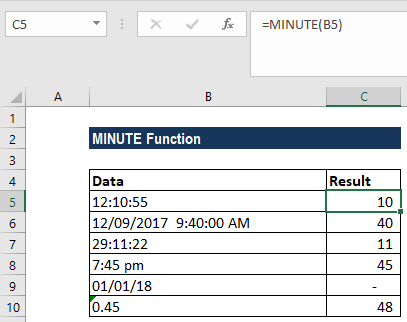# MINUTE Function

Returns the minute component of a time

## What is the MINUTE Function?

The MINUTE function is categorized under Excel DATE/TIME functions. The function returns the minute component of a time as a number between 0-59. For example, it will return 49 for 1.49 PM.

### Formula

=MINUTE(serial_number)

The MINUTE function uses only one argument:

Serial_number (required argument) – The time that contains the minute we want to find.

The argument can be entered as:

1. A decimal (serial number) representation of an Excel time
2. A text representation of a time
3. A reference to a cell containing a time
4. A time value returned from another Excel function or formula

### How to use the MINUTE Function in Excel?

As a worksheet function, MINUTE can be entered as part of a formula in a cell of a worksheet. To understand the uses of the MINUTE function, let’s consider an example:

#### Example 1

Let’s see how this function works using the following inputs:The results in Excel are shown in the screenshot below:In case we get an error, we need to remember that Excel stores dates and times as serial numbers. For example, the date January 1, 2000, 12:00 PM is equal to the serial number 32526.5 in Excel. To check that Excel correctly recognizes a date or time, we can temporarily format the date as a number.

### A few notes about the MINUTE Function:

1. Time values are a portion of a date value and represented by a decimal number (for example, 12:00 PM is represented as 0.5 because it is half of a day).
2. #VALUE! error – Occurs when the given serial_number is not a valid Excel time.

Thanks for reading CFI’s guide to important Excel functions! By taking the time to learn and master these functions, you’ll significantly speed up your financial analysis. To learn more, check out these additional CFI resources:

• Excel Functions for Finance